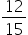Mathematics
Easy

Question

# The denominator for the given fractionis _____________.

## The correct answer is: 15

### Given fraction.To find - the denominator of the given fraction.The part below the line in a fraction is called denominator.Hence, the denominator is 15

The denominator of the given fractionis 15.

### Related Questions to study#### With Turito Foundation.#### Get an Expert Advice From Turito.